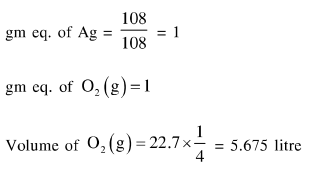# Solve this followingQuestion:

$108 \mathrm{~g}$ of silver (molar mass $108 \mathrm{~g} \mathrm{~mol}^{-1}$ ) is deposited at cathode from $\mathrm{AgNO}_{3}(\mathrm{aq})$ solution by a certain quantity of electricity. The volume (in L) of oxygen gas produced at $273 \mathrm{~K}$ and 1 bar pressure from water by the same quantity of electricity is

Solution: